﻿ Bond Graphs of the Electrical RLC Circuit

### Bond Graphs of the Electrical RLC Circuit

Darina Hroncová, Alexander GmiterkoOPEN ACCESSPEER-REVIEWED

## Bond Graphs of the Electrical RLC Circuit

Darina Hroncová1,, Alexander Gmiterko1

1Department of applied mechanics and mechatronics, Technical University of Košice / Faculty of mechanical engineering, Košice, Slovakia

### Abstract

The work shows the use of Bond Graph formalism for modeling dynamic systems. As an example a electrical model is solved by this approach at the level of its physical behavior. In contrast with the classical method, where the equations for individual components are created first and then the simulation scheme is derived on their basis, the described method uses the reverse procedure. In this paper the method of generation of system equations is discussed. From a bond graph diagram of the system, using a step-by-step procedure, system equations may be generated. As a starting point a model of a simple electrical RLC circuit consisting of a resistor, an inductor, and a capacitor is taken. The differential equations describing the dynamics of the system are obtained in terms of the states of the system.

### At a glance: Figures

1234
Prev Next

• Hroncová, Darina, and Alexander Gmiterko. "Bond Graphs of the Electrical RLC Circuit." American Journal of Mechanical Engineering 1.7 (2013): 318-323.
• Hroncová, D. , & Gmiterko, A. (2013). Bond Graphs of the Electrical RLC Circuit. American Journal of Mechanical Engineering, 1(7), 318-323.
• Hroncová, Darina, and Alexander Gmiterko. "Bond Graphs of the Electrical RLC Circuit." American Journal of Mechanical Engineering 1, no. 7 (2013): 318-323.

 Import into BibTeX Import into EndNote Import into RefMan Import into RefWorks

### 1. Introduction

The concept of bond graphs was originated by Paynter (1961). The idea was further developed by Karnopp and Rosenberg in their textbooks (1968, 1975, 1983, 1990), such that it could be used in practice (Thoma, 1975; Van Dixhoorn, 1982). By means of the formulation by Breedveld (1984, 1985) of a framework based on thermodynamics, bond-graph model description evolved to a systems theory [1-7].

A causal bond graph contains all information to derive the set of state equations. The procedure to derive these equations is covered by bond graph software like Enport (Rosenberg, 1974), MS1 (Lorenz, 1997), CAMP (Granda, 1985), and 20-SIM (Broenink, 1990, 1995, 1997, 1999; Broenink and Kleijn, 1999). Therefore, in practice, generation of equation need not be done by hand. However, we discuss the generation of equations to indicate what exactly has to be done.

### 2. Bond Graph of the Electrical System

The language of bond graphs aspires to express general class physical systems through power interactions. The factors of power i.e., effort and flow, have different interpretations in different physical domains. Yet, power can always be used as a generalized coordinate to model coupled systems residing in several energy domains.

2.1. Description of the Model

To demonstrate the bond graph methodology as an example an electrical model of RLC system is analyzed in Figure 1 a). An RLC circuit (or LCR circuit) is an electrical circuit consisting of a resistor, an inductor, and a capacitor. The RLC part of the name is due to those letters being the usual electrical symbols for resistance, inductance and capacitance respectively .

Figure 1. Electrical system: a) electrical model of RLC system; b) reference voltage uref with positive direction; c) efforts (voltages) with unique names u1, u2, u3
2.2. Bond Graph Construction

We have discussed the basic bond-graph elements and the bond, so we can transform a domain-dependent ideal-physical model, written in domain-dependent symbols, into a bond graph. For this transformation, there is a systematic procedure, which is presented here. This electrical system contains a voltage source effort SE (SE:uz), a resistor R (R:R1, R:R2), an inductor I (I:L1, I:L2,) and a capacitor C (C:1/C).

Step 1 – Determine which physical domains exist in the system and identify all basic elements like C (capacitor), I (inductor), R (resistor), SE (source of the effort), SF (source of the flow), TF (transformer) and GY (gyrator).

This system contains a electrical domain part with the inductance L of the inductor (I:L), the resistance R of the resistor (R:R) and a capacity of the capacitor C (C:C). Voltage uz is considered as an effort source (SE: uz).

Step 2 – Indicate in the ideal-physical model per domain a reference source – effort voltage uref (reference voltage with positive direction) in Figure 1 b).

The references are indicated in the ideal physical model: the ideal velocity uref = 0.

Step 3 – Identify all other efforts (voltages) and give them unique names u1, u2, u3, Figure 1 c).

Step 4 – Draw these efforts (electrical: voltages), graphically by 0-junctions in Figure 2 a).

Step 5 – Identify all effort differences (electrical: voltage = effort) needed to connect the ports of all elements enumerated in step 1 to the junction structure. When checking all ports of the elements found in step 1 linear voltage differences, u12 and u23 are identified: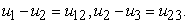(1)

Step 6 – Construct the voltages differences using a 1-junction and draw them as such in the graph in Figure 2 b).

The junction structure is now ready and the elements can be connected.

Step 7 – Connect the port of all elements found at step 1 with the 1-junction of the corresponding efforts or efforts differences in Figure 3 a).

Step 8 – Simplify the resulting graph by applying the simplification rules in Figure 3 b) and in Figure 4 a).

A junction between two bonds can be left out, if the bonds have a through power direction (one bond incoming, the other outgoing). A bond between two the same junctions can be left out, and the junctions can join into one junction. Two separately constructed identical effort or flow differences can join into one effort or flow difference.

Step 9 – Determine the signal direction and causality in Figure 4 b). Causality establishes the cause and effect relationships between the factors of power.

In bond graphs, the inputs and the outputs are characterized by the causal stroke. The causal stroke indicates the direction in which the effort signal is directed (by implication, the end of the bond that does not have a causal stroke is the end towards which the flow signal is directed).

There are two ways of describing an element's behavior (e.g. effort in, flow out vs. flow in, effort out) as different causal forms. Note that the two alternative causal forms may, in general, require quite different mathematical operations. The causal form we use, i.e. which variable we select as input and which we select as output, can make a lot of difference. For example, the required mathematical operations may be well defined in one causal form, but not defined at all in the other.

The causal bond graph of this system can be derived, in which the inputs and the outputs are characterized by the causal stroke. This is the starting point, from which we continue toward the differential equations describing the dynamics of the system.

2.3. Expansion to Block Diagrams

The equations are derived in the steps described below.

A causal bond graph in Figure 5 b) can be expanded into a block diagram in three steps.

Step 1 – All node symbols are encircled in Figure 7 a).

Step 2 – All bonds are expanded into bilateral signal flows according to the assigned causality in Figure 7 b).

Step 3 – All constitutive relations of each node are written into block diagram form, according to the assigned causality of each port; one-junction is represented by a signal-node for the flows and a summation for the efforts in Figure 8.

All signals entering a summation resulting from a junction are given a sign corresponding to the half-arrow direction: if, while traveling from causal input to causal output, the bond orientation does not change (this does not exclude an orientation opposite to the signal direction), then a plus sign is added representing a positive contribution to the summation; by contrast if the bond orientation does change, then a minus sign is added representing a negative contribution to the summation. In principle, a complete block diagram is obtained at this point.

The procedure to obtain a signal flow graph is completely analogous to the above procedure as all operations represented by blocks, including the signs of the summations, are combined as much as possible and then written next to an edge, while all summations become nodes, as signal nodes can be distinguished from signal summation points by observing the signal directions (signal node has only one input, summation has only one output).

#### Table 1. Element in block diagramDownload asPowerPoint Slide

Veiw figureView current table in a new window

2.4. Generation of the Equations

The state equations derived from a bond graph diagram in Figure 10 a) and in Figure 10 b) are: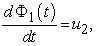(2)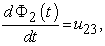(3)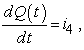(4)

where state variables Φ1(t), Φ2(t) and Q(t) are magnetic flux and electric charge respectively.

Now we rewrite variable parameters from Figure 9 and Figure 10 to equations: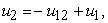(5)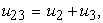(6)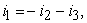(7)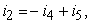(8)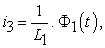(9)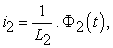(10)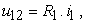(11)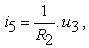(12)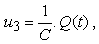(13)

After substitution we get: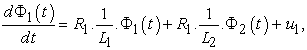(14)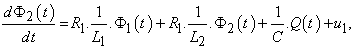(15)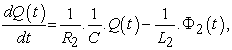(16)

and in matrix form: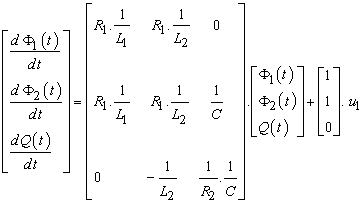(17)

By choosing different state variables i electric current and u3 electric charge, after substitution the state equations are in following form: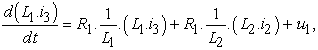(18)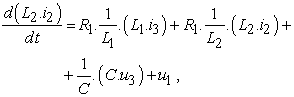(19)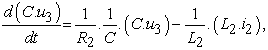(20)

and finally: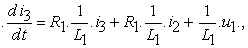(21)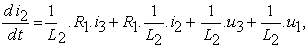(22)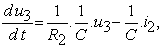(23)

or in matrix form: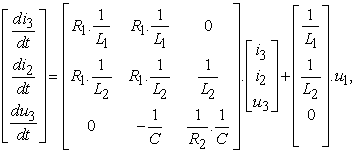(24)

The equation can be solved in Matlab/Simulink for example [8-13].

2.5. State Space Equation in Matlab/Simulink

Solution of the non-homogenous system of differential equations of a mechanical system with two degrees of freedom in Figure 11, is done in Matlab/Simulink using State-Space. The most general state-space representation of a linear system with u(t) inputs, y(t) outputs and n state variables is written in the following form: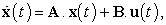(25)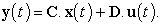(26)

where A, B, C, D are the respective matrices of the mechanical system defined as follows:

We determine the matrix and block diagram in Matlab/Simulink shown in Figure 11:

### 3. Conclusion

Bond graphs represent a convenient tool for physical system analysis. We presented a method to systematically build a bond graph starting from an ideal physical model. Causal analysis gives, besides the computational direction of the signals at the bonds, also information about the correctness of the model. A practical example of an electrical model is presented as the application of this methodology.

The differential equations describing the dynamics of the system in terms of the states of the system were derived from a bond graph diagram of a simple electrical system. The results correspond with equations obtained using traditional method, where the equations for individual components are created first and then the simulation scheme is derived on their basis, although the described method uses the reverse procedure.

However, manual derivation of equations for larger systems is not all that simple. For instance, in some cases the derivation may lead to formation of so called algebraic loops. Similarly, complexities and errors of various types, like causal loops, power loops and differential causalities may exist in the model of a system.

### Acknowledgement

This work was supported by grant projects VEGA No. 1/0289/11, VEGA No. 1/1205/12 and grant projects VEGA No. 1/0937/12 and APVV-0091-11.

### References

  Breedveld, P., C., Rosenberg, R., C., Zhou, T., 1991. Bibliography of Bond Graph Theory and Application, Journal of the Franklin Institute, 328(5/6), p. 1067-1109.In article CrossRef  Breedveld, P., C. Modeling and Simulation of Dynamic Systems Using Bond Graphs, http://eolss.net.In article  Broenink, J., F. Introduction to Physical Systems Modelling with Bond Graphs, http://www.ce.utwente.nl.In article  Virgala, , Frankovský, P., Kenderová, M.: Friction Effect Analysis of a DC Motor. In: American Journal of Mechanical Engineering. 2013. Vol. 1, no. 1 (2013), p. 1-5In article  Karnopp, D., C., Margolis, D., L., Rosenberg, R., C., 2005. Modeling and simulation of mechatronic systems. John Willey & Sons, Inc., .In article  Horáček, P., 1999. Systems and models, Nakladatelství ČVUT, Praha, Česko.In article  Gmiterko, A., Šarga, P., Hroncová, D., 2012. Mechatronika I, Technická univerzita Košice, .In article  Hroncová, D., 2012. Studies of compatible software tools for abstract and concrete design of mechatronic systems and linking them for the purpose of logical and physical modeling, Dissertation thesis, .In article  Karban, P., 2006. Výpočty a simulace v programech Matlab a Simulink. Computer Press, Brno, Česko.In article  Gmiterko, A., 2004. Mechatronics, Emilenia, .In article  Vittek, J., 1997. Matlab pre elektrické pohony, Nakladateľstvo Žilinskej univerzity, Žilina, Slovensko, p. 35-36.In article  Ogatha, K., 1978. System Dynamics. Prentice Hall, Inc., Cliffs, .In article  Delyová, I., Frankovský, P., Hroncová, D., 2011. Kinematics analysis of movement of a point of a simple mechanism, In: Modelling of mechanical and mechatronic systems 2011, The 4th International conference, TU Košice, Herľany, Slovakia.In article